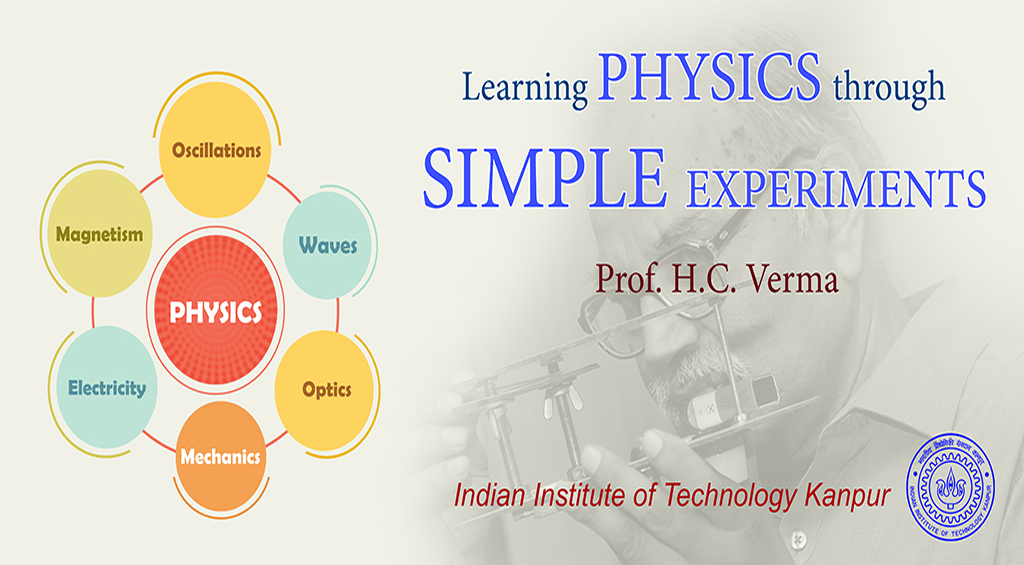##### Introduction

This course covers some basic principles of Physics and describes them through few simple experiments. Sometimes the phenomena is shown first, then in order to understand and analyze it, the theory is explained. In some cases it is the reverse.
The discussion is roughly at +2 and UG first year level but the experiments could be an educative experience for students from grade 9 onwards. Most of these experiments throw some challenge to understand it, so even the well trained persons up to Postgraduate level will find it interesting!

##### Core target groups are

Physics teachers teaching in schools from grade 9 to 12

Physics teachers teaching in colleges at B Sc, B.Tech level

Students from grade 9 to BSc/B.Tech

Others interested in enjoying Physics displayed by Nature in day-to-day life

##### Optics

• Fermat’s Principle
• Diffraction and Interference
• Refraction
• Polarization
• Dispersion
• Scattering
• Prisms and Lenses
• Reflection

##### Mechanics

• Classification of forces
• Rolling
• Non inertial frames and pseudo forces
• Angular momentum, Moment of inertia, Rigid body rotation
• Rotating frames, Centrifugal and coriolis force
• Friction and Card-Coin experiment
• Conical Pendulum

##### Electricity/Magnetism

• Circular motion of charge in magnetic field
• Diamagnetic and paramagnetic materials
• Force on a current in a magnetic field
• Curie Temperature
• B-field of a bar magnet and forces on a magnetic material in this field
• Misconceptions on poles
• Non conservative Electric Field
• Role of inhomogeneity in force on a magnet
• Lenz’s law and eddy currents

##### Oscillations and waves

• Stable equilibrium and oscillations
• Complexity of Simple pendulum
• Damped / Forced oscillations
• Waves on a slinky, a string
• Similarity between mechanical and L-C-R oscillations
• Wave speed, dependence on tension and linear density
• Resonance
• Standing waves on strings and on springs